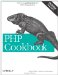# Recipe 4.6. Changing Array Size

#### 4.6.1. Problem

You want to modify the size of an array, either by making it larger or smaller than its current size.

#### 4.6.2. Solution

Use array_pad( ) to make an array grow:

`// start at three \$array = array('apple', 'banana', 'coconut'); // grow to five \$array = array_pad(\$array, 5, '');`

Now , count(\$array) is 5, and the last two elements, \$array and \$array, contain the empty string.

To reduce an array, you can use array_splice( ):

`// no assignment to \$array array_splice(\$array, 2);`

This removes all but the first two elements from \$array.

#### 4.6.3. Discussion

Arrays aren't a predeclared size in PHP, so you can resize them on the fly.

To pad an array, use array_pad( ). The first argument is the array to be padded. The next argument is the size and direction you want to pad. To pad to the right, use a positive integer; to pad to the left, use a negative one. The third argument is the value to be assigned to the newly created entries. The function returns a modified array and doesn't alter the original.

Here are some examples:

`// make a four-element array with 'dates' to the right \$array = array('apple', 'banana', 'coconut'); \$array = array_pad(\$array, 4, 'dates'); print_r(\$array); Array (      => apple      => banana      => coconut      => dates ) // make a six-element array with 'zucchinis' to the left \$array = array_pad(\$array, -6, 'zucchini'); print_r(\$array); Array (      => zucchini      => zucchini      => apple      => banana      => coconut      => dates )`

Be careful: array_pad(\$array, 4, 'dates') makes sure an \$array is at least four elements long; it doesn't add four new elements. In this case, if \$array was already four elements or larger, array_pad( ) would return an unaltered \$array.

Also, if you declare a value for a fourth element, \$array:

`\$array = array('apple', 'banana', 'coconut'); \$array = 'dates';`

you end up with a four-element array with indexes 0, 1, 2, and 4:

`Array (      => apple      => banana      => coconut      => dates )`

PHP essentially turns this into an associative array that happens to have integer keys.

The array_splice( ) function, unlike array_pad( ), has the side effect of modifying the original array. It returns the spliced-out array. That's why you don't assign the return value to \$array. However, like array_pad( ), you can splice from either the right or left. So calling array_splice( ) with a value of -2 chops off the last two elements from the end:

`// make a four-element array \$array = array('apple', 'banana', 'coconut', 'dates'); // shrink to three elements array_splice(\$array, 3); // remove last element, equivalent to array_pop() array_splice(\$array, -1); // only remaining fruits are apple and banana print_r(\$array); Array (      => apple      => banana )`

Documentation on array_pad( ) at http://www.php.net/array-pad and array_splice( ) at http://www.php.net/array-splice .PHP Cookbook: Solutions and Examples for PHP Programmers
ISBN: 0596101015
EAN: 2147483647
Year: 2006
Pages: 445

Similar book on Amazon